# Rate of convergence

## Definition

The rate of convergence for a convergent sequence describes how quickly the terms of the sequence converge to the limit. We discuss some cases below.

For the definitions below, the sequence is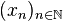$(x_n)_{n \in \mathbb{N}}$ and the limit is$L$. In symbols, we have: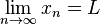$\lim_{n \to \infty} x_n = L$

### Steadily linear, superlinear, and sublinear convergence

Suppose the following holds: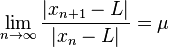$\lim_{n \to \infty} \frac{|x_{n+1} - L|}{|x_n - L|} = \mu$

Clearly,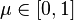$\mu \in [0,1]$ (if$\mu$ were greater than 1, the sequence couldn't converge). We have three cases:

•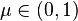$\mu \in (0,1)$, i.e.,$0 < \mu < 1$: In this case, the sequence$(x_n)$ converges to$L$ linearly, and the constant$\mu$ is called the rate of (linear) convergence. The use of the term "linear" signifies that the next error term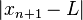$|x_{n+1} - L|$ is approximately a linear function of the preceding error term$|x_n - L|$.
•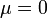$\mu = 0$: In this case, the sequence$(x_n)$ converges to$L$ superlinearly. In other words, a function that would approximate the behavior of the next error term in terms of the previous error term would decay faster than a linear function.
•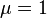$\mu = 1$: In this case, the sequence$(x_n)$ converges to$L$ sublinearly. In other words, a function that would describe the next error term in terms of the previous one would decay slower than a linear function.

### Convergence of a power function form

Suppose$q > 1$ is a real number. We say that the sequence$(x_n)$ converges to a limit$L$ with order of convergence$q$ if the following holds: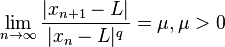$\lim_{n \to \infty} \frac{|x_{n+1} - L|}{|x_n - L|^q} = \mu, \mu > 0$

In other words, if we were to try to construct a function that approximately predicted$|x_{n+1} - L|$ in terms of$|x_n - L|$, the order of zero of a the function at zero would be$q$. Note that convergence of this form is superlinear.

Two special cases:

•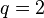$q = 2$, in which case we say the sequence converges quadratically or has quadratic convergence.
•$q = 3$, in which case we say the sequence converges cubically or has cubic convergence.

### Logarithmic convergence

For further information, refer: logarithmic convergence

If the sequence converges sublinearly, and further: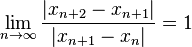$\lim_{n \to \infty} \frac{|x_{n+2} - x_{n+1}|}{|x_{n+1} - x_n|} = 1$

then we say that the convergence is logarithmic. This use of terminology differs somewhat from the others, because it is not the case that we can use a logarithmic function to approximate the description of$|x_{n+1} - L|$ in terms of$|x_n - L|$. (So what's the rationale of the term?)

We say that a sequence$(x_n)$ has R-convergence of a particular type to a limit$L$ if we can find a sequence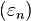$(\varepsilon_n)$ such that, for all$n$:$|x_n - L| < \varepsilon_n$
and the sequence$\varepsilon_n$ has convergence to 0 of that type.